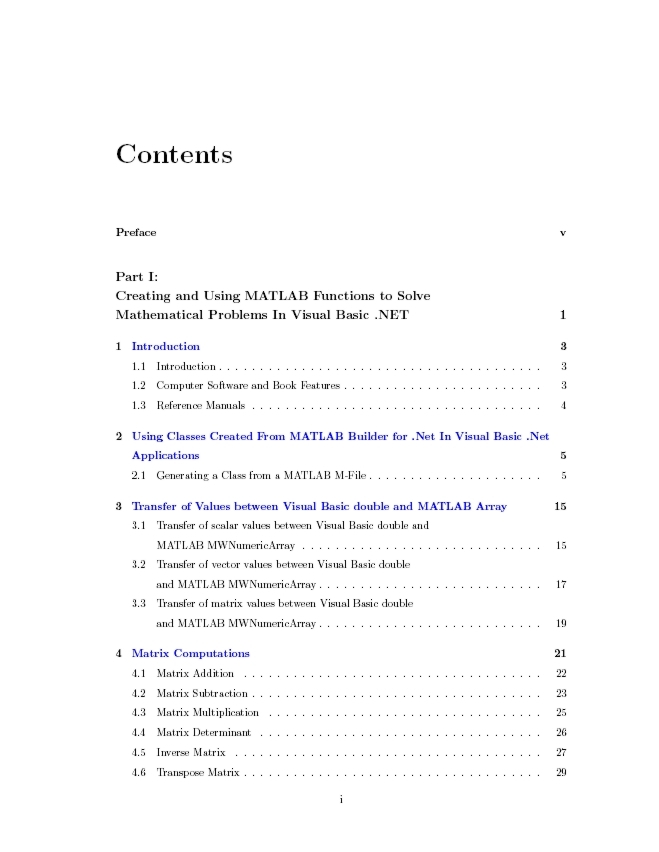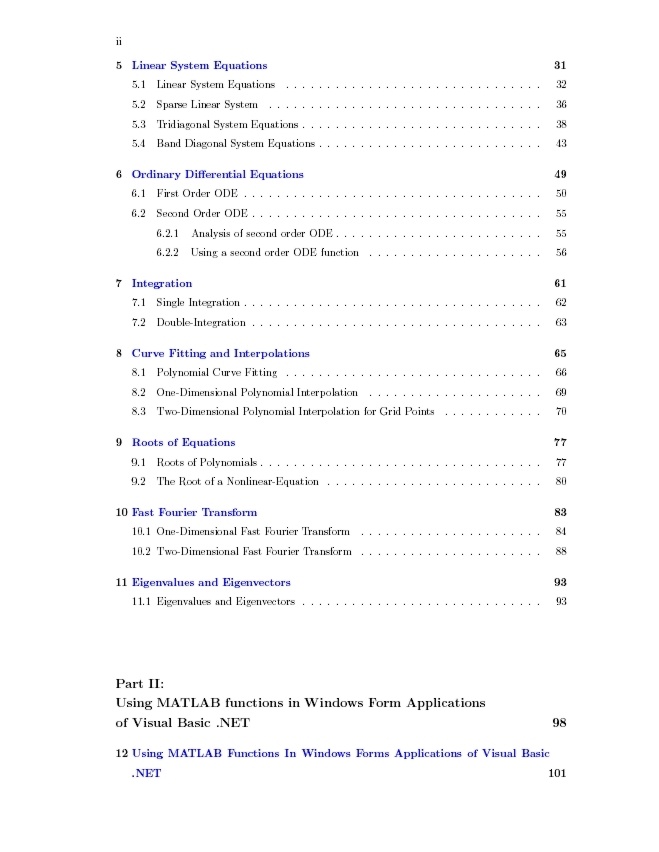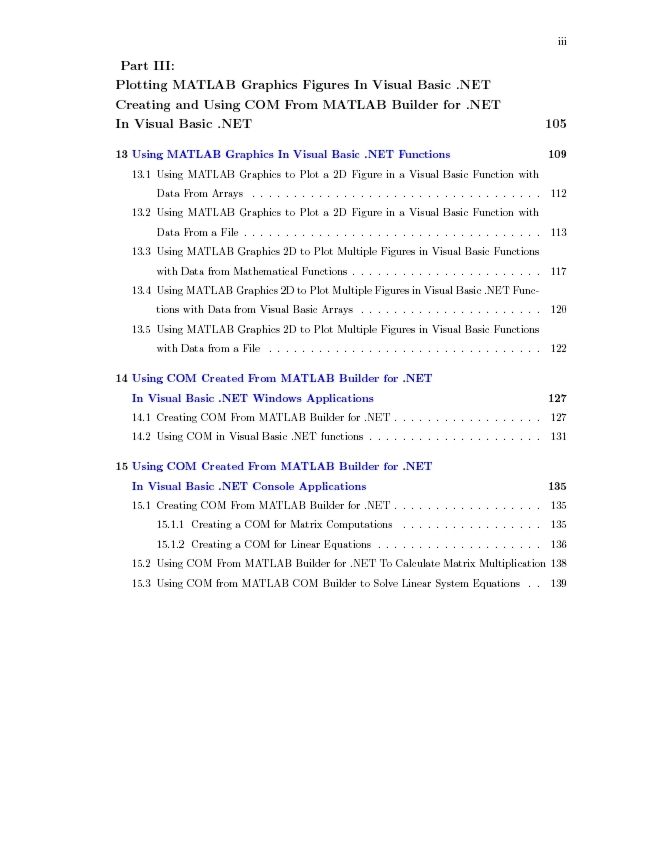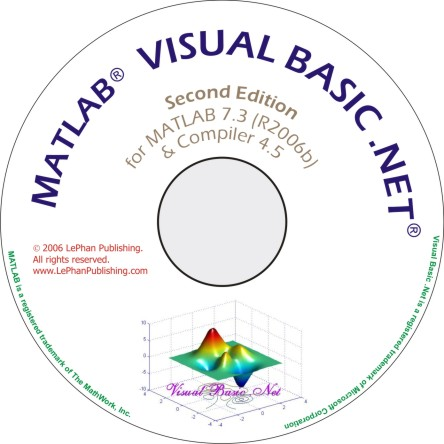MATLABÛ   Visual Basic .NET Û    Book

Second Edition for MATLAB 7.3, MATLAB Builder for .NET 2.0, and MATLAB Compiler 4.5

MATLAB Visual Basic .NET Book illustrates the works between Visual Basic .NET programming and MATLAB. The  book describes very detail how to use MATLAB built-in functions in Visual Basic .NET applications. If you are a Visual Basic .NET programmer and want to use the quality mathematical built-in functions in your Visual Basic .NET applications to solve mathematical problems in Visual Basic .NET programming code, then this book is what you're looking for.

The features of this book are designed to handle following topics:

1. Visual Basic .NET functions call MATLAB built-in functions in the class created from MATLAB M-files to solve the mathematical problems.

2. Visual Basic .NET Windows Applications uses MATLAB built-in functions.

3. Visual Basic .NET functions plot figures from MATLAB Graphics.

4. Visual Basic .NET functions use COM generated from MATLAB M-files.

This MATLAB Visual Basic .NET Book is an ebook in the pdf format on CD-Rom which also includes the completed Visual Basic .NET code examples of all chapters. The example codes are developed, compiled, and tested in  MATLAB 7.3 (R2006), MATLAB Compiler 4.5, MATLAB Builder for .NET 2.1. The examples are working on scalars, vectors, and matrixes which are inputs/outputs of functions for every application. In addition, the example codes are portable and presented in the step-by-step method, therefore you can easily reuse the codes or write your own codes following the step-by-step procedure.

The MATLAB Visual Basic .NET Book is a great support for Visual Basic .NET programmers who are using MATLAB built-in functions to develop applications and solutions. Using the combination of both tools, Visual Basic .NET and MATLAB, you have the best tool in your hand to develop and solve your  technical problems. This book is reviewed by MATLAB staff and promoted at MATLAB Book Program.

MATLAB and MATLAB Compiler are registered trademarks of The MathWork, Inc.
Microsoft, Windows, and Microsoft Visual Basic .NET are